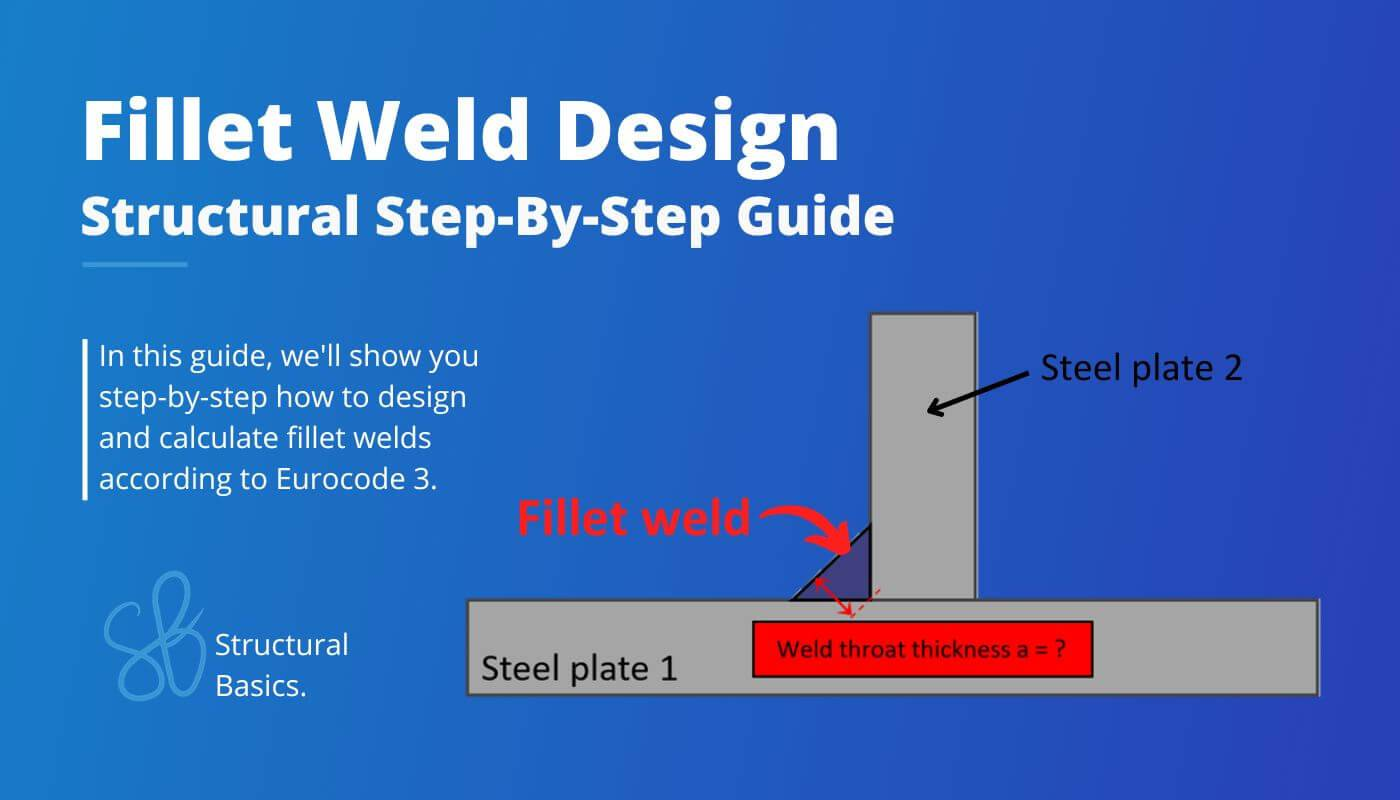# Fillet Weld Design {2023 Engineering Guide}Fillet welds are one of the most common types of welds.

In this article, we’ll show you step-by-step with an example structure how to design and calculate them.

So let’s get started.

## Design Resistance Of Fillet Welds

According to Eurocode EN 1993-1-8 4.5.3.1, either the directional method or the simplified method should be used to calculate the bearing capacity of fillet welds.

We’ll explain both in the following.

### Directional Method

In the directional method, the forces acting on the weld are translated into force components parallel and transverse to its longitudinal axis and normal and transverse to its throat plane (EN 1993-1-8 4.5.3.2).

The fillet weld verifies if the following 2 criteria are satisfied (EN 1993-1-8 (4.1):

Criteria 1:

$$\sqrt{\sigma_{90}^2 + 3 (\tau_0^2 + \tau_{90}^2)} \le \frac{f_u}{\beta_w \gamma_{M2}}$$

Criteria 2:

$$\sigma_{90} \le 0.9 \frac{f_u}{\gamma_{M2}}$$

Ok, so now let’s break down its components.

##### Stresses $\sigma_{90}$, $\tau_{90}$ and $\tau_{0}$

The stresses in the weld are calculated from the internal forces acting in the plate:

• Bending moment M
• Normal force N
• Shear force V

$$\sigma_{90} = \frac{N}{2 \cdot l_{eff} \cdot a \cdot \sqrt{2}} + \frac{M}{2 \cdot l_{eff}^2 \cdot a/6 \cdot \sqrt{2}}$$

$$\tau_{90} = \sigma_{90}$$

$$\tau_{0} = \frac{V}{2 \cdot l_{eff} \cdot a}$$

With,

Let’s continue by checking out the steel property parameters that we also need for the verification. 💯💯

### Simplified Method

The second method that Eurocode recommends is the simplified method. In textbooks, it’s said that this method is conservative.

In this method, the following criteria has to be fulfilled:

$$F_{w.Ed} \le \frac{f_u \cdot a}{\sqrt{3} \cdot \beta_w \cdot \gamma_{M2}}$$

With

For the following weld that is only exposed to a tension force, $F_{w.Ed}$ is calculated as

$$F_{w.Ed} = \frac{T}{b}$$

## Example Calculation With Directional Method

For the following example structure, a fillet weld makes most sense, because the plates are easy to weld from both sides.

We calculate the fillet weld for the following internal forces:

## Example Calculation With Simplified Method

We’ll use the same example structure as for the directional method.

The internal forces are the same as for the directional method.

### Verification

As we can see, this method has a higher utilization as the directional method.

## Conclusion

Et voilà, the fillet weld is verified and dimensioned. 💯💯

That was a quick verification, wasn’t it?

If you are new to structural design, then check out more of our design tutorials where you can also learn how to design other steel, concrete and wood elements such as

But now, I would like to hear from you: Have you already designed a fillet weld in university or at your work? Tell us a bit about the structure, as we all want to learn from each other.